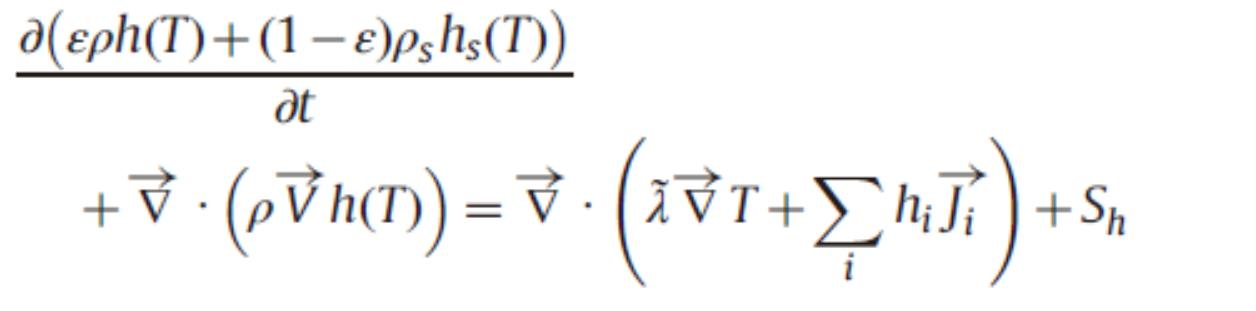# how do fluent calculate energy equation?

Hi all,

I have a question : how fluent calculate energy equation? I put the energy equation in enthalpy change formulate below:the author mentioned both enthalpy and specific heat are temperature dependent. I have defined temperature function of specific heat. However, I cann't see why enthalpy should be defined with UDF. Does the author mean enthalpy is a function of specific heat and specific heat is function of temperature. Then enthalpy is function of temperature indirectly. Therefore, I just need to define temperature dependent function of specific heat in fluent?

Best.

Weiqiang.

• edited May 2019
Enthalpy is always temperature dependent. You can define temperature dependent specific heat.
• Do you mean I have to define temperature-dependent enthalpy of species by myself or fluent can calculate the temperature-dependent enthalpy automatically?

Best.

Weiqiang

• edited May 2019
Fluent does it automatically. Enthalpy is integral of Cp wrt dT plus ss enthalpy all is mass fraction weighted so the sum goes over all species.
• thanks very much!

Best regards.

Weiqiang